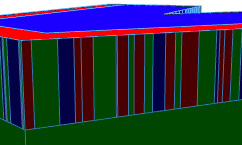# CGA Randomize split inner section / repeats

139
2
02-14-2020 08:46 AMNew Contributor

Hello Guys,

Is there a way to change the random value for each iteration during split?

opTopFloor -->

split(x) { rand(1.0, 5.0) : colorRed X.

| rand(1.0, 5.0) : colorGreen X.

| rand(1.0, 5.0) : colorBlue X.}*

it appears the rand() is evaluated once, instead of each iteration.

What I would need is that each iteration {}* would provide a new random number

doable?

Tx

2 RepliesNew Contributor

I was able to achieve this using this, but there is got a better wayopTopFloor2 -->

split(x) { rand(1.0, 7.0) : split(x) { rand(0.0, 1.0) : colorRed X.

| rand(0.0, 1.0) : colorGreen X.

| rand(0.0, 1.0) : colorBlue X.}* }*byEsri Regular Contributor

You are correct.  The random number is evaluated, and the repeat split is applied using that number for all repeated parts.

``split(x) { rand(0,1): X. }*‍‍// Let's say rand(0,1) gives us 0.3, then the split above is equivalent to the following:split(x) { 0.3: X. }*‍‍‍‍‍‍‍‍‍‍‍‍‍‍‍‍‍‍‍‍‍‍‍‍‍‍‍‍‍‍``

In order to split into parts that have random sizes for each part, you can't use a repeat split.  Instead, you should use a recursive split which recursively splits out one part at a time with a random size.  Here is an example which recursively splits a shape into parts X that have random sizes between [0,1].

``RecursiveSplit -->     case scope.sz<1:          X     else:          split(x) { rand(0,1): X                   | ~1: RecursiveSplit }‍‍‍‍‍‍‍‍‍‍‍‍‍‍‍‍‍‍‍‍‍‍‍‍‍‍‍``

Actually, since the split will just stop safely when there isn't any geometry left to split, then there is no need to even have the case statement as the end condition.  The following is sufficient.

``RecursiveSplit -->     split(x) { rand(0,1): X.              | ~1: RecursiveSplit }‍‍‍‍‍‍‍``

In your case, to alternate red, green, and blue colors, one way to do this would be:

``RecursiveSplit3 -->     split(x) { rand(0,1): color(1,0,0) X.              | rand(0,1): color(0,1,0) X.              | rand(0,1): color(0,0,1) X.              | ~1: RecursiveSplit3 }‍‍‍‍‍``